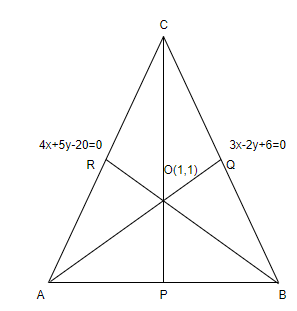Courses
Courses for Kids
Free study material
Free LIVE classes
More# Let the equations of two sides of a triangle be $3x-2y+6=0$ and $4x+5y-20=0$. If theorthocentre of this triangle is at $(1,1)$, then the equation of its third side is(a) $122y-26x-1675=0$(b) $26x+61y+1675=0$(c) $122y+26x+1675=0$(d) $26x-122y-1675=0$Verified
330.6k+ views
Hint: Find the slope of the given two lines and use this slope and given orthocentre to find the coordinates of the triangle.

The equations of the sides of a triangle given in the questions are,
$3x-2y+6=0$ and $4x+5y-20=0$
The orthocentre of a triangle is the point where all the altitudes intersect. Its coordinates are given
in the question as $(1,1)$. Draw a triangle ABC with all the given data as shown below,
Consider the line AC. The slope of the line can be obtained by rearranging the equation as,
\begin{align} & 4x+5y-20=0 \\ & 5y=20-4x \\ & y=-\dfrac{4}{5}x+4 \\ \end{align}
Comparing it with the general equation, $y=mx+c$, we have the slope as $-\dfrac{4}{5}$. The slope
of perpendicular lines is related as $m\times n=-1$. Now since the line BR is perpendicular to the
line AC, the slope of BR would be $\dfrac{5}{4}$.
The line BR has slope $\dfrac{5}{4}$ and passes through the point $(1,1)$. The equation of a line
passing through point $({{x}_{1}},{{y}_{1}})$ and having slope $m$ is given by,$y-{{y}_{1}}=m(x- {{x}_{1}})$. The equation of the line BR can hence be obtained as,
\begin{align} & y-1=\dfrac{5}{4}(x-1) \\ & 4(y-1)=5(x-1) \\ & 4y-4=5x-5 \\ & 5x-4y-1=0 \\ \end{align}
Next, we have to consider line BC. The slope of the line can be obtained by rearranging the equation
as,
\begin{align} & 3x-2y+6=0 \\ & -2y=-6-3x \\ & y=\dfrac{3}{2}x+3 \\ \end{align}
Comparing it with the general equation, $y=mx+c$, we have the slope as $\dfrac{3}{2}$. The slope
of perpendicular lines is related as $m\times n=-1$. Now since the line AQ is perpendicular to the
line BC, the slope of AQ would be $-\dfrac{2}{3}$.
The line AQ has slope $-\dfrac{2}{3}$ and passes through the point $(1,1)$. The equation of a line
passing through point $({{x}_{1}},{{y}_{1}})$ and having slope $m$ is given by,$y-{{y}_{1}}=m(x- {{x}_{1}})$. The equation of the line AQ can hence be obtained as,
\begin{align} & y-1=-\dfrac{2}{3}(x-1) \\ & 3(y-1)=-2(x-1) \\ & 3y-3=-2x+2 \\ & 2x+3y-5=0 \\ \end{align}
The vertex A is passing through both lines AC and AQ. So, we can get the coordinates of vertex A by
solving the equations $4x+5y-20=0$ and $2x+3y-5=0$. Multiplying the equation $2x+3y-5=0$ by 2
and subtracting from the equation $4x+5y-20=0$, we get,
\dfrac{\begin{align} & 4x+5y-20=0 \\ & -4x-6y+10=0 \\ \end{align}}{\begin{align} & -y-10=0 \\ & y=-10 \\ \end{align}}
Substituting the value of $y$ in $2x+3y-5=0$, we get,
\begin{align} & 2x+(3\times -10)-5=0 \\ & 2x-30-5=0 \\ & 2x=35 \\ & x=\dfrac{35}{2} \\ \end{align}
Therefore, the coordinates of A are $\left( \dfrac{35}{2},-10 \right)$.
The vertex B is passing through both lines BC and BR. So, we can get the coordinates of vertex B by
solving the equations $3x-2y+6=0$ and $5x-4y-1=0$ . Multiplying the equation $3x-2y+6=0$ by 2
and subtracting the equation $5x-4y-1=0$ from it, we get,
\dfrac{\begin{align} & 6x-4y+12=0 \\ & -5x+4y+1=0 \\ \end{align}}{\begin{align} & x+13=0 \\ & x=-13 \\ \end{align}}
Substituting the value of $x$ in $3x-2y+6=0$, we get,
\begin{align} & (3\times -13)-2y+6=0 \\ & -39-2y+6=0 \\ & -2y=33 \\ & y=-\dfrac{33}{2} \\ \end{align}
Therefore, the coordinates of B are $\left( -13,-\dfrac{33}{2} \right)$.
The equation of a line passing through two points $({{x}_{1}},{{y}_{1}})$ and $({{x}_{2}},{{y}_{2}})$ is
given by,
\begin{align} & y-{{y}_{1}}=m(x-{{x}_{1}}) \\ & \Rightarrow y-{{y}_{1}}=\dfrac{{{y}_{2}}-{{y}_{1}}}{{{x}_{2}}-{{x}_{1}}}\times (x-{{x}_{1}}) \\ \end{align}
The equation of line AB passing through points $\left( \dfrac{35}{2},-10 \right)$ and $\left( -13,- \dfrac{33}{2} \right)$ can hence be found as,
$\left( y+10 \right)=\dfrac{\left( -\dfrac{33}{2}+10 \right)}{\left( -13-\dfrac{35}{2} \right)}\times \left( x-\dfrac{35}{2} \right)$
Taking the LCM of the terms in the numerator and denominator,
\begin{align} & y+10=\dfrac{-\dfrac{13}{2}}{-\dfrac{61}{2}}\times \left( x-\dfrac{35}{2} \right) \\ & y+10=\dfrac{13}{61}\times \left( x-\dfrac{35}{2} \right) \\ & 61(y+10)=13\left( x-\dfrac{35}{2} \right) \\ & 61y+610=13x-\dfrac{455}{2} \\ \end{align}
Multiplying both sides by 2,
\begin{align} & 122y+1220=26x-455 \\ & 26x-122y-1675=0 \\ \end{align}
Therefore, the required equation of the straight line is $26x-122y-1675=0$.
We get option (d) as the correct answer.

Note: There is one more way to approach this problem. The coordinates of the vertices A and B can
be taken as $\left( p,\dfrac{20-4p}{5} \right)$ and $\left( q,\dfrac{3+6q}{2} \right)$ by assuming
$x$ as $p$ and $q$ respectively in the equations $4x+5y-20=0$and $3x-2y+6=0$. Then we can
formulate the equations using the slope of the lines and solve for $p$ and $q$. The equation of AB
can then be obtained.Last updated date: 24th May 2023
Total views: 330.6k
Views today: 6.88k